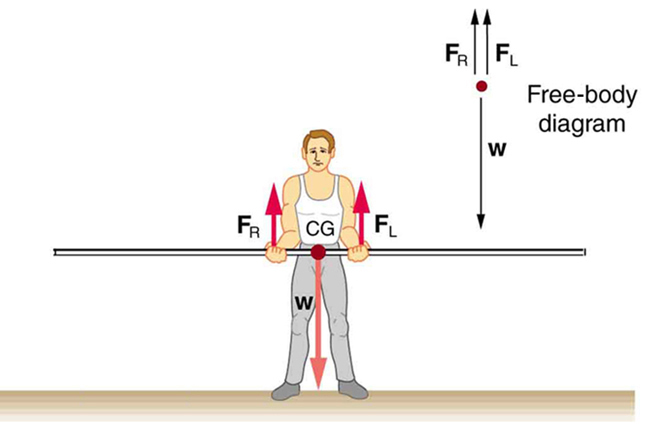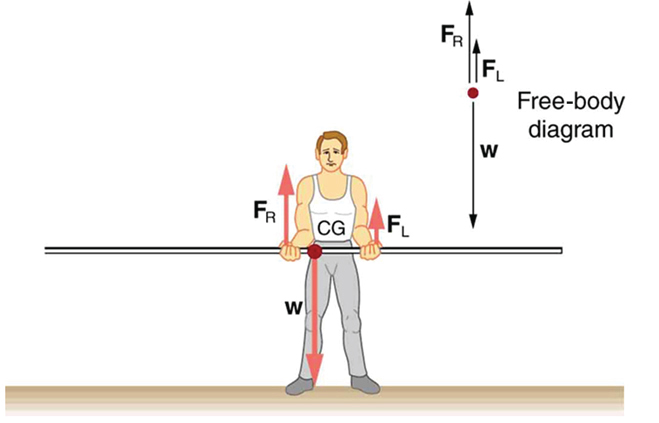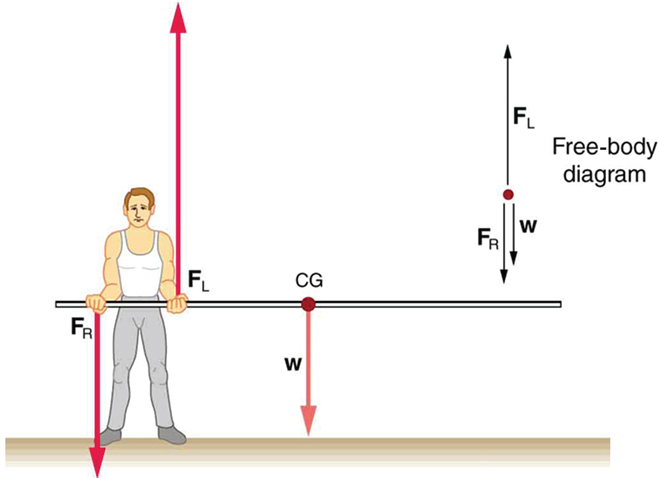# 8.1 Applications of statics, including problem-solving strategies  (Page 2/3)

 Page 2 / 3

Similar observations can be made using a meter stick held at different locations along its length.A pole vaulter holds a pole horizontally with both hands.A pole vaulter is holding a pole horizontally with both hands. The center of gravity is near his right hand.A pole vaulter is holding a pole horizontally with both hands. The center of gravity is to the left side of the vaulter.

If the pole vaulter holds the pole as shown in [link] , the situation is not as simple. The total force he exerts is still equal to the weight of the pole, but it is not evenly divided between his hands. (If ${F}_{L}={F}_{R}$ , then the torques about the cg would not be equal since the lever arms are different.) Logically, the right hand should support more weight, since it is closer to the cg. In fact, if the right hand is moved directly under the cg, it will support all the weight. This situation is exactly analogous to two people carrying a load; the one closer to the cg carries more of its weight. Finding the forces ${F}_{L}$ and ${F}_{R}$ is straightforward, as the next example shows.

If the pole vaulter holds the pole from near the end of the pole ( [link] ), the direction of the force applied by the right hand of the vaulter reverses its direction.

## What force is needed to support a weight held near its cg?

For the situation shown in [link] , calculate: (a) ${F}_{R}$ , the force exerted by the right hand, and (b) ${F}_{L}$ , the force exerted by the left hand. The hands are 0.900 m apart, and the cg of the pole is 0.600 m from the left hand.

Strategy

[link] includes a free body diagram for the pole, the system of interest. There is not enough information to use the first condition for equilibrium $\text{(net}\phantom{\rule{0.25em}{0ex}}F=0$ ), since two of the three forces are unknown and the hand forces cannot be assumed to be equal in this case. There is enough information to use the second condition for equilibrium $\text{(net}\phantom{\rule{0.25em}{0ex}}\tau =0\text{)}$ if the pivot point is chosen to be at either hand, thereby making the torque from that hand zero. We choose to locate the pivot at the left hand in this part of the problem, to eliminate the torque from the left hand.

Solution for (a)

There are now only two nonzero torques, those from the gravitational force ( ${\tau }_{\text{w}}$ ) and from the push or pull of the right hand ( ${\tau }_{R}$ ). Stating the second condition in terms of clockwise and counterclockwise torques,

$\text{net}\phantom{\rule{0.25em}{0ex}}{\tau }_{\text{cw}}=\text{–net}\phantom{\rule{0.25em}{0ex}}{\tau }_{\text{ccw}}\text{.}$

or the algebraic sum of the torques is zero.

Here this is

${\tau }_{R}={\mathrm{–\tau }}_{\text{w}}$

since the weight of the pole creates a counterclockwise torque and the right hand counters with a clockwise torque. Using the definition of torque, $\tau =\text{rF}\phantom{\rule{0.25em}{0ex}}\text{sin}\phantom{\rule{0.25em}{0ex}}\theta$ , noting that $\theta =90º$ , and substituting known values, we obtain

$\left(0\text{.}\text{900 m}\right)\left({F}_{R}\right)=\left(0\text{.600 m}\right)\left(\mathit{mg}\right)\text{.}$

Thus,

$\begin{array}{lll}{F}_{R}& =& \left(0.667\right)\left(\text{5.00 kg}\right)\left(9.80\phantom{\rule{0.25em}{0ex}}{\text{m/s}}^{2}\right)\\ & =& \text{32.7 N.}\end{array}$

Solution for (b)

The first condition for equilibrium is based on the free body diagram in the figure. This implies that by Newton’s second law:

${F}_{L}+{F}_{R}–\text{mg}=0$

From this we can conclude:

${F}_{L}+{F}_{R}=w=\text{mg}$

Solving for ${F}_{L}$ , we obtain

$\begin{array}{lll}{F}_{L}& =& \mathit{mg}-{F}_{R}\\ & =& \mathit{mg}-\text{32}\text{.}7 N\\ & =& \left(\text{5.00 kg}\right)\left(\text{9.80}\phantom{\rule{0.25em}{0ex}}{\text{m/s}}^{2}\right)-\text{32.7 N}\\ & =& \text{16.3 N}\end{array}$

Discussion

${F}_{L}$ is seen to be exactly half of ${F}_{R}$ , as we might have guessed, since ${F}_{L}$ is applied twice as far from the cg as ${F}_{R}$ .

If the pole vaulter holds the pole as he might at the start of a run, shown in [link] , the forces change again. Both are considerably greater, and one force reverses direction.

## Take-home experiment

This is an experiment to perform while standing in a bus or a train. Stand facing sideways. How do you move your body to readjust the distribution of your mass as the bus accelerates and decelerates? Now stand facing forward. How do you move your body to readjust the distribution of your mass as the bus accelerates and decelerates? Why is it easier and safer to stand facing sideways rather than forward? Note: For your safety (and those around you), make sure you are holding onto something while you carry out this activity!

## Phet explorations: balancing act

Play with objects on a teeter totter to learn about balance. Test what you've learned by trying the Balance Challenge game.Balancing Act

## Summary

• Statics can be applied to a variety of situations, ranging from raising a drawbridge to bad posture and back strain. We have discussed the problem-solving strategies specifically useful for statics. Statics is a special case of Newton’s laws, both the general problem-solving strategies and the special strategies for Newton’s laws, discussed in Problem-Solving Strategies , still apply.

## Conceptual questions

When visiting some countries, you may see a person balancing a load on the head. Explain why the center of mass of the load needs to be directly above the person’s neck vertebrae.

## Problems&Exercises

To get up on the roof, a person (mass 70.0 kg) places a 6.00-m aluminum ladder (mass 10.0 kg) against the house on a concrete pad with the base of the ladder 2.00 m from the house. The ladder rests against a plastic rain gutter, which we can assume to be frictionless. The center of mass of the ladder is 2 m from the bottom. The person is standing 3 m from the bottom. What are the magnitudes of the forces on the ladder at the top and bottom?

In [link] , the cg of the pole held by the pole vaulter is 2.00 m from the left hand, and the hands are 0.700 m apart. Calculate the force exerted by (a) his right hand and (b) his left hand. (c) If each hand supports half the weight of the pole in [link] , show that the second condition for equilibrium $\text{(net}\phantom{\rule{0.25em}{0ex}}{\tau }_{}=\text{0)}$ is satisfied for a pivot other than the one located at the center of gravity of the pole. Explicitly show how you follow the steps in the Problem-Solving Strategy for static equilibrium described above.

#### Questions & Answers

Is there any normative that regulates the use of silver nanoparticles?
Damian Reply
what king of growth are you checking .?
Renato
What fields keep nano created devices from performing or assimulating ? Magnetic fields ? Are do they assimilate ?
Stoney Reply
why we need to study biomolecules, molecular biology in nanotechnology?
Adin Reply
?
Kyle
yes I'm doing my masters in nanotechnology, we are being studying all these domains as well..
Adin
why?
Adin
what school?
Kyle
biomolecules are e building blocks of every organics and inorganic materials.
Joe
anyone know any internet site where one can find nanotechnology papers?
Damian Reply
research.net
kanaga
sciencedirect big data base
Ernesto
Introduction about quantum dots in nanotechnology
Praveena Reply
what does nano mean?
Anassong Reply
nano basically means 10^(-9). nanometer is a unit to measure length.
Bharti
do you think it's worthwhile in the long term to study the effects and possibilities of nanotechnology on viral treatment?
Damian Reply
absolutely yes
Daniel
how to know photocatalytic properties of tio2 nanoparticles...what to do now
Akash Reply
it is a goid question and i want to know the answer as well
Maciej
characteristics of micro business
Abigail
for teaching engĺish at school how nano technology help us
Anassong
Do somebody tell me a best nano engineering book for beginners?
s. Reply
there is no specific books for beginners but there is book called principle of nanotechnology
NANO
what is fullerene does it is used to make bukky balls
Devang Reply
are you nano engineer ?
s.
fullerene is a bucky ball aka Carbon 60 molecule. It was name by the architect Fuller. He design the geodesic dome. it resembles a soccer ball.
Tarell
what is the actual application of fullerenes nowadays?
Damian
That is a great question Damian. best way to answer that question is to Google it. there are hundreds of applications for buck minister fullerenes, from medical to aerospace. you can also find plenty of research papers that will give you great detail on the potential applications of fullerenes.
Tarell
what is the Synthesis, properties,and applications of carbon nano chemistry
Abhijith Reply
Mostly, they use nano carbon for electronics and for materials to be strengthened.
Virgil
is Bucky paper clear?
CYNTHIA
carbon nanotubes has various application in fuel cells membrane, current research on cancer drug,and in electronics MEMS and NEMS etc
NANO
so some one know about replacing silicon atom with phosphorous in semiconductors device?
s. Reply
Yeah, it is a pain to say the least. You basically have to heat the substarte up to around 1000 degrees celcius then pass phosphene gas over top of it, which is explosive and toxic by the way, under very low pressure.
Harper
Do you know which machine is used to that process?
s.
how to fabricate graphene ink ?
SUYASH Reply
for screen printed electrodes ?
SUYASH
What is lattice structure?
s. Reply
of graphene you mean?
Ebrahim
or in general
Ebrahim
in general
s.
Graphene has a hexagonal structure
tahir
On having this app for quite a bit time, Haven't realised there's a chat room in it.
Cied
what is biological synthesis of nanoparticles
Sanket Reply
how did you get the value of 2000N.What calculations are needed to arrive at it
Smarajit Reply
Privacy Information Security Software Version 1.1a
Good

### Read also:

#### Get the best Algebra and trigonometry course in your pocket!

Source:  OpenStax, College physics arranged for cpslo phys141. OpenStax CNX. Dec 23, 2014 Download for free at http://legacy.cnx.org/content/col11718/1.4
Google Play and the Google Play logo are trademarks of Google Inc.

Notification Switch

Would you like to follow the 'College physics arranged for cpslo phys141' conversation and receive update notifications?ByBy